### Home > CAAC > Chapter 14 > Lesson 14.3.1.4 > Problem3-57

3-57.
1. Solve each equation below for x. Refer back to problem 3-30 for examples, if necessary. Homework Help ✎

1. 2(x+3) = 64

2. 8x = 46

3.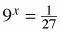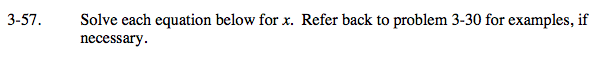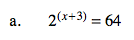64 = 26

2(x + 3) = 26

x + 3 = 6

x = 3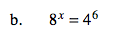Do 8 and 4 have a common base?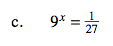$\text{Notice: }\frac{1}{27}=3^{-3}$